Скачать презентацию Verification Methodology Based on Algorithmic State Machines and

34cf51e2c403fc13a178a859f3e5355d.ppt

• Количество слайдов: 24Verification Methodology Based on Algorithmic State Machines and Cycle-Accurate Contract Specifications Sergey Frenkel 1 and Alexander Kamkin 2 1 Institute of Informatics Problems of the Russian Academy of Sciences E-mail: [email protected] ru 2 Institute for System Programming of the Russian Academy of Sciences E-mail: [email protected] ru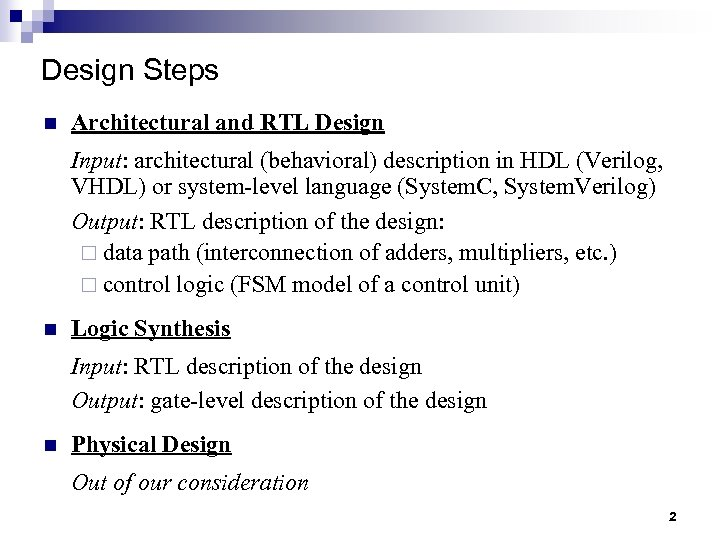Design Steps n Architectural and RTL Design Input: architectural (behavioral) description in HDL (Verilog, VHDL) or system-level language (System. C, System. Verilog) Output: RTL description of the design: ¨ data path (interconnection of adders, multipliers, etc. ) ¨ control logic (FSM model of a control unit) n Logic Synthesis Input: RTL description of the design Output: gate-level description of the design n Physical Design Out of our consideration 2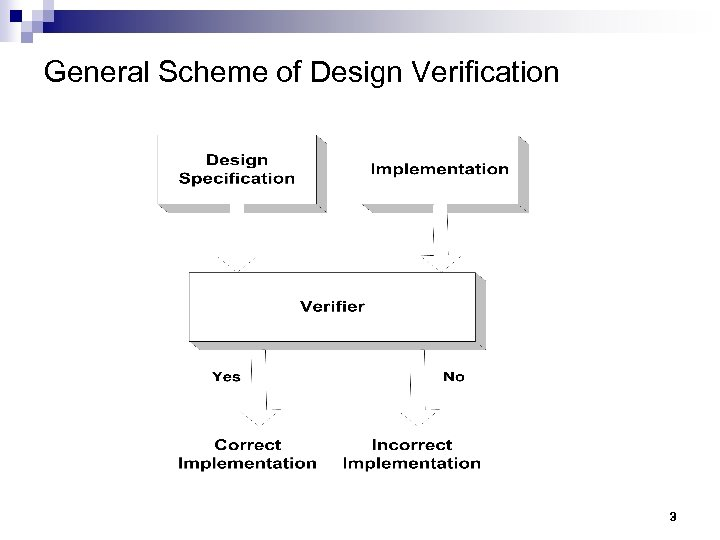General Scheme of Design Verification 3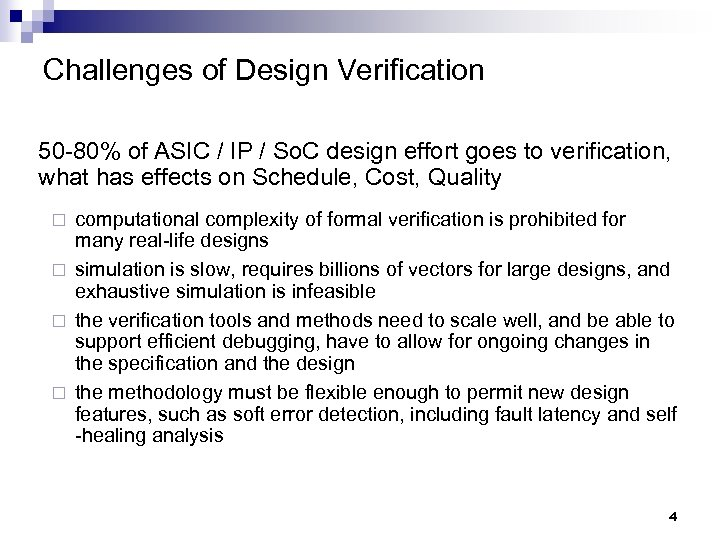Challenges of Design Verification 50 -80% of ASIC / IP / So. C design effort goes to verification, what has effects on Schedule, Cost, Quality computational complexity of formal verification is prohibited for many real-life designs ¨ simulation is slow, requires billions of vectors for large designs, and exhaustive simulation is infeasible ¨ the verification tools and methods need to scale well, and be able to support efficient debugging, have to allow for ongoing changes in the specification and the design ¨ the methodology must be flexible enough to permit new design features, such as soft error detection, including fault latency and self -healing analysis ¨ 4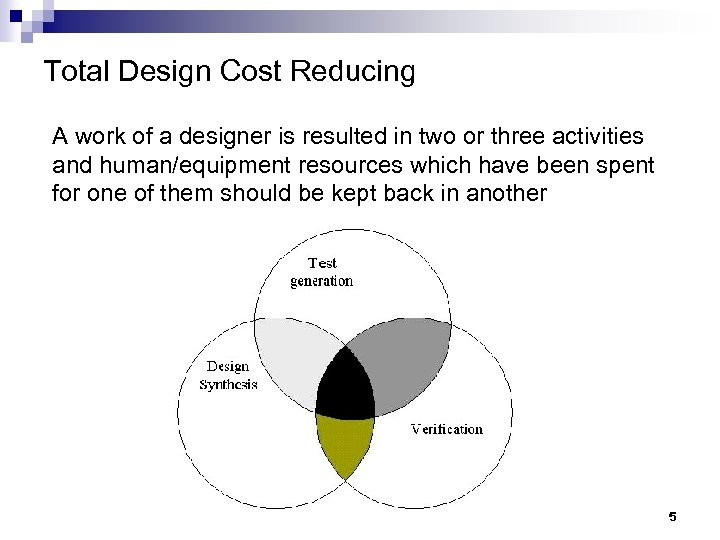Total Design Cost Reducing A work of a designer is resulted in two or three activities and human/equipment resources which have been spent for one of them should be kept back in another 5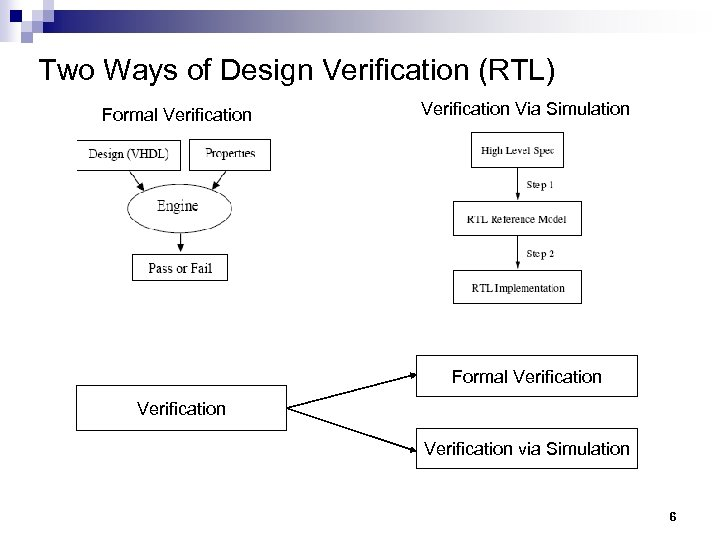Two Ways of Design Verification (RTL) Formal Verification Via Simulation Formal Verification via Simulation 6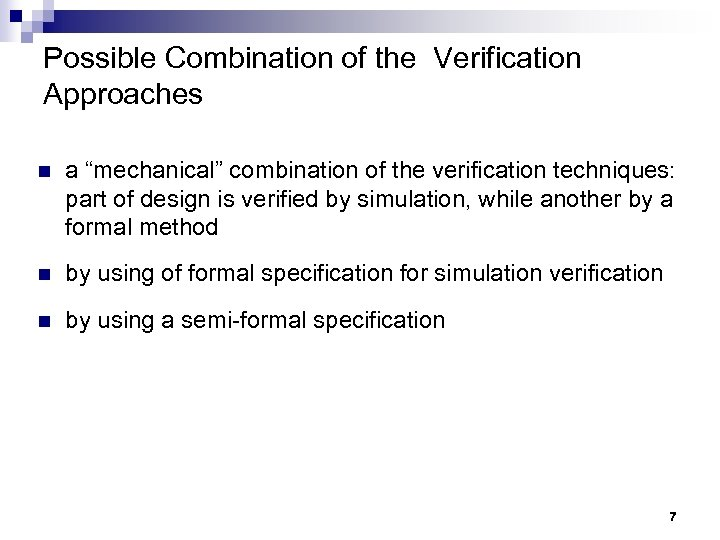Possible Combination of the Verification Approaches n a “mechanical” combination of the verification techniques: part of design is verified by simulation, while another by a formal method n by using of formal specification for simulation verification n by using a semi-formal specification 7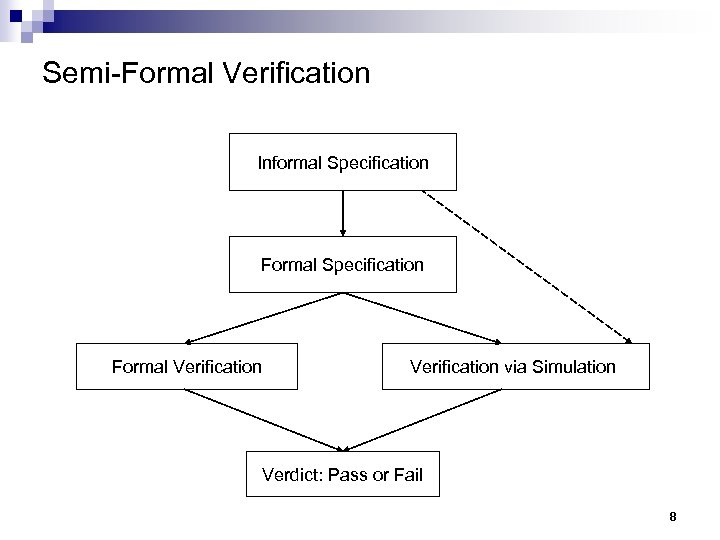Semi-Formal Verification Informal Specification Formal Verification via Simulation Verdict: Pass or Fail 8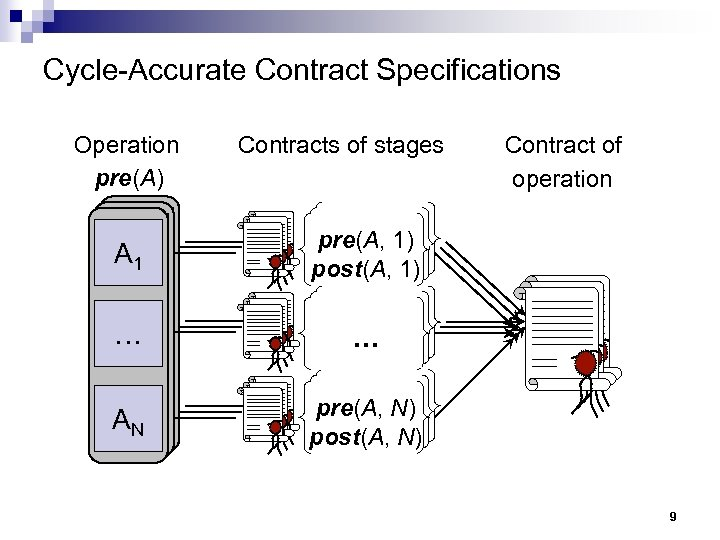Cycle-Accurate Contract Specifications Operations pre(A) Contracts of stages A A A 111 pre(A, 1) post(A, 1) … … … A AN ANN Contract of Contracts of operations pre(A, N) post(A, N) 9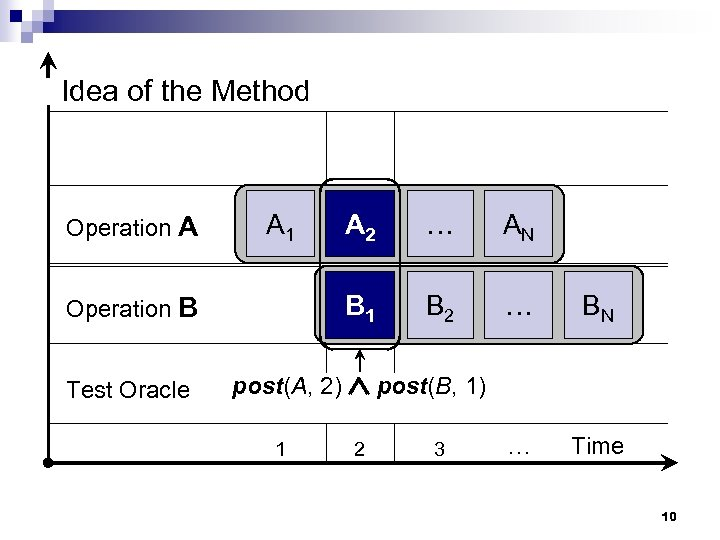Idea of the Method A 2 … AN Operation B B 1 B 2 … BN Test Oracle post(A, 2) post(B, 1) … Time Operation A A 1 1 2 3 10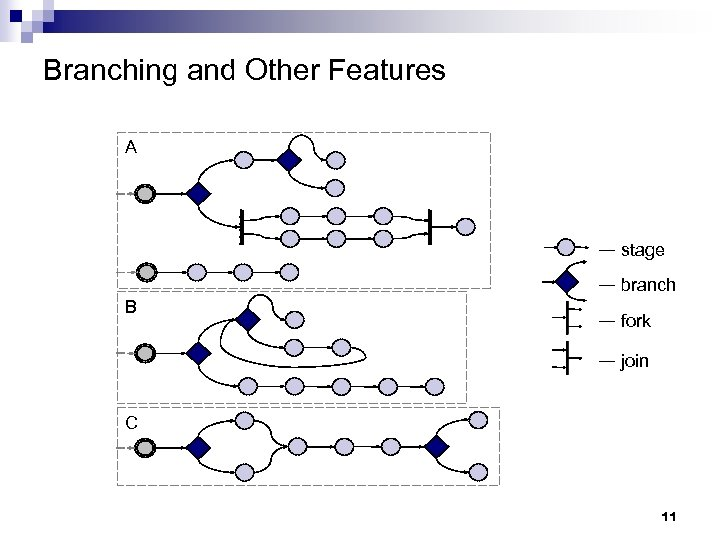Branching and Other Features A — stage B — branch — fork — join C 11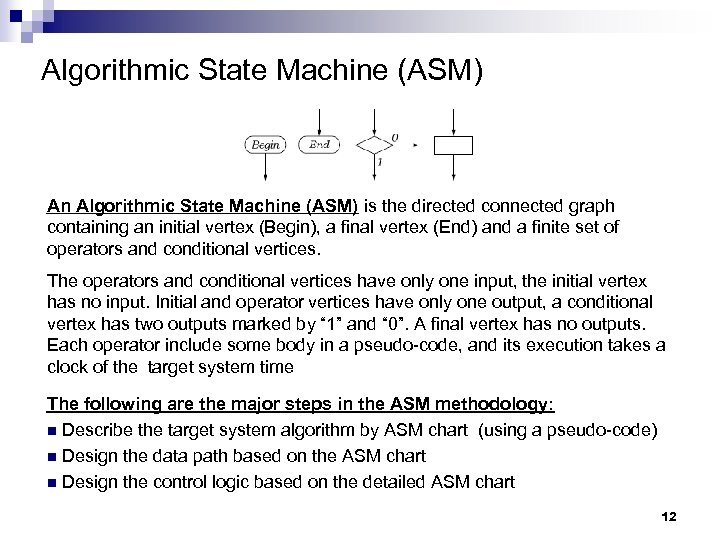Algorithmic State Machine (ASM) An Algorithmic State Machine (ASM) is the directed connected graph containing an initial vertex (Begin), a final vertex (End) and a finite set of operators and conditional vertices. The operators and conditional vertices have only one input, the initial vertex has no input. Initial and operator vertices have only one output, a conditional vertex has two outputs marked by “ 1” and “ 0”. A final vertex has no outputs. Each operator include some body in a pseudo-code, and its execution takes a clock of the target system time The following are the major steps in the ASM methodology: n Describe the target system algorithm by ASM chart (using a pseudo-code) n Design the data path based on the ASM chart n Design the control logic based on the detailed ASM chart 12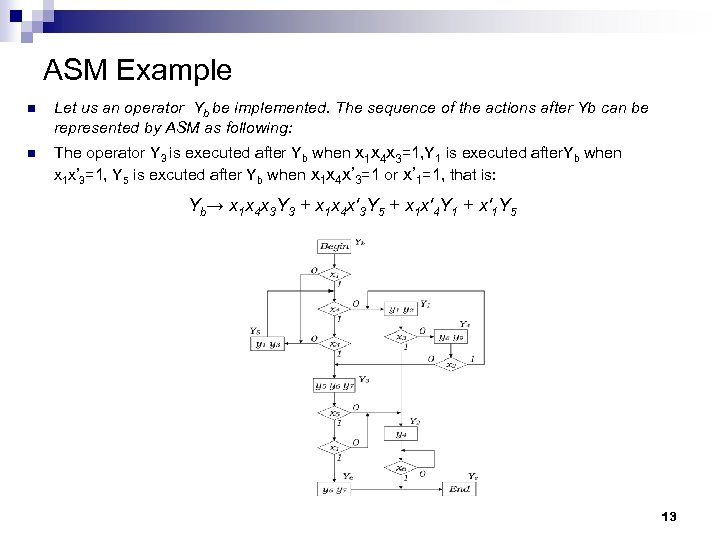ASM Example n Let us an operator Yb be implemented. The sequence of the actions after Yb can be represented by ASM as following: n The operator Y 3 is executed after Yb when x 1 x 4 x 3=1, Y 1 is executed after. Yb when x 1 x’ 3=1, Y 5 is excuted after Yb when x 1 x 4 x’ 3=1 or x’ 1=1, that is: Yb→ x 1 x 4 x 3 Y 3 + x 1 x 4 x'3 Y 5 + x 1 x'4 Y 1 + x'1 Y 5 13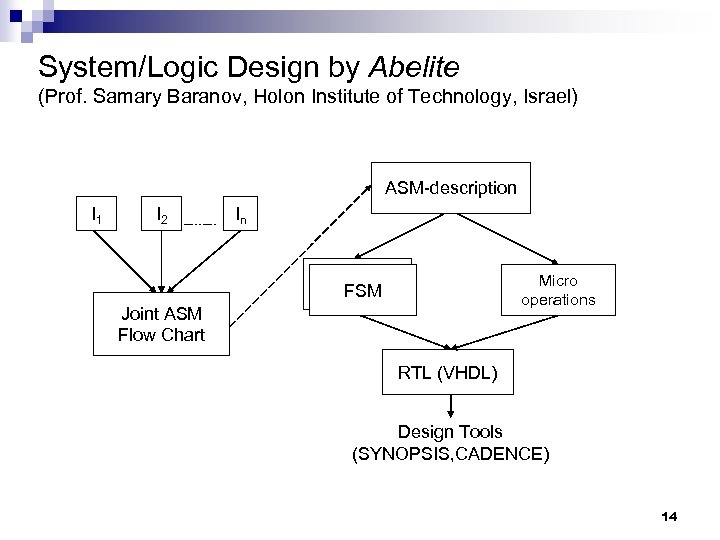System/Logic Design by Abelite (Prof. Samary Baranov, Holon Institute of Technology, Israel) ASM-description I 1 I 2 In Micro operations FSM Joint ASM Flow Chart RTL (VHDL) Design Tools (SYNOPSIS, CADENCE) 14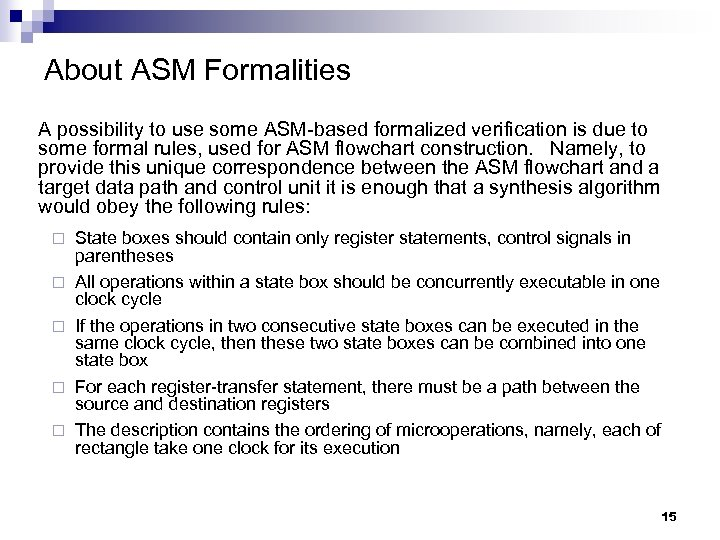About ASM Formalities A possibility to use some ASM-based formalized verification is due to some formal rules, used for ASM flowchart construction. Namely, to provide this unique correspondence between the ASM flowchart and a target data path and control unit it is enough that a synthesis algorithm would obey the following rules: ¨ ¨ ¨ State boxes should contain only register statements, control signals in parentheses All operations within a state box should be concurrently executable in one clock cycle If the operations in two consecutive state boxes can be executed in the same clock cycle, then these two state boxes can be combined into one state box For each register-transfer statement, there must be a path between the source and destination registers The description contains the ordering of microoperations, namely, each of rectangle take one clock for its execution 15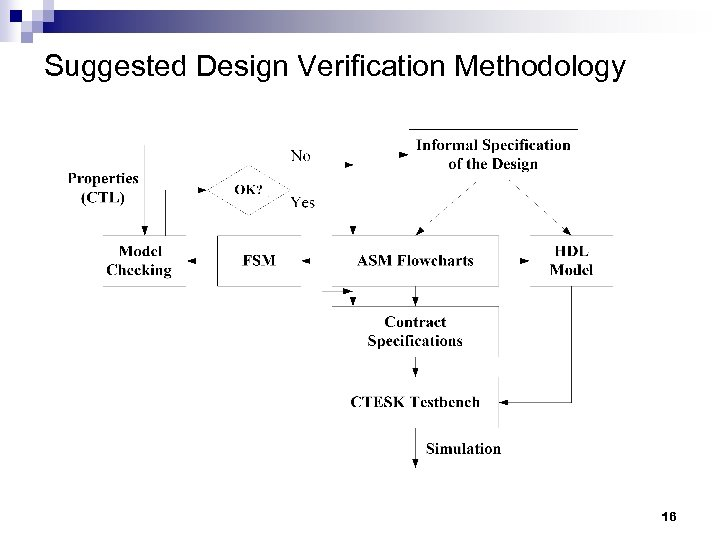Suggested Design Verification Methodology 16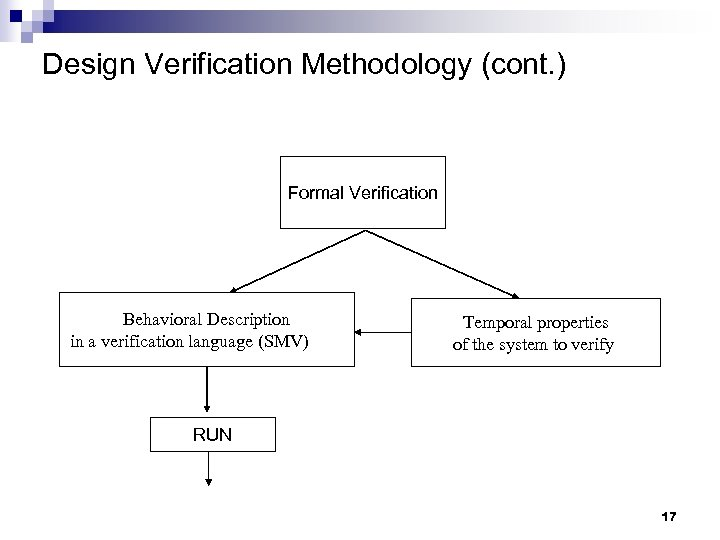Design Verification Methodology (cont. ) Formal Verification Behavioral Description in a verification language (SMV) Temporal properties of the system to verify RUN 17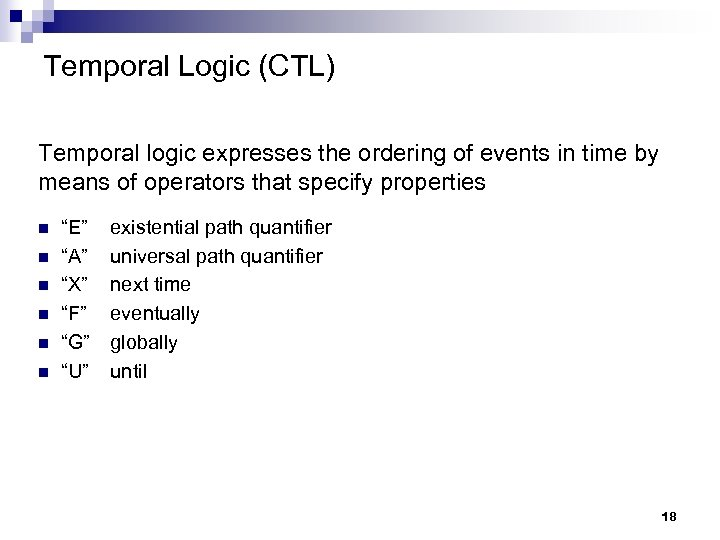Temporal Logic (CTL) Temporal logic expresses the ordering of events in time by means of operators that specify properties n n n “E” “A” “X” “F” “G” “U” existential path quantifier universal path quantifier next time eventually globally until 18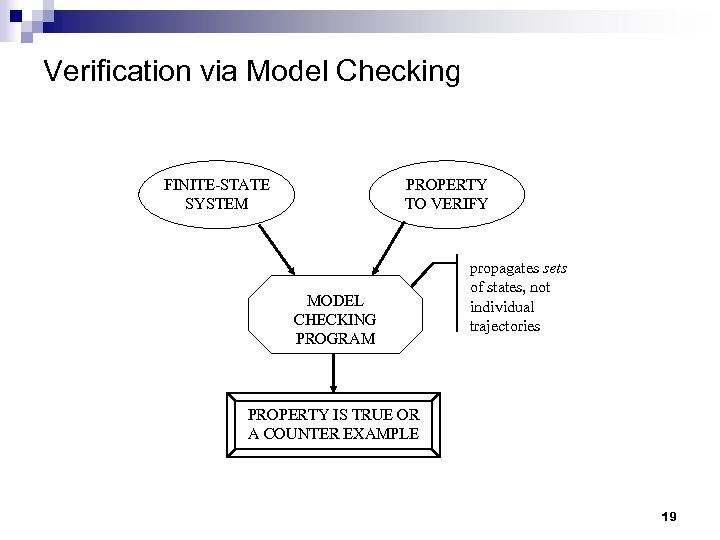Verification via Model Checking FINITE-STATE SYSTEM PROPERTY TO VERIFY MODEL CHECKING PROGRAM propagates sets of states, not individual trajectories PROPERTY IS TRUE OR A COUNTER EXAMPLE 19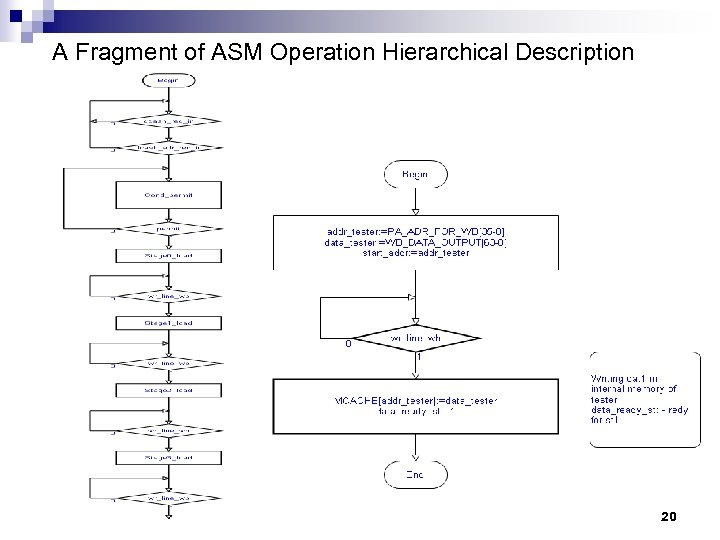A Fragment of ASM Operation Hierarchical Description 20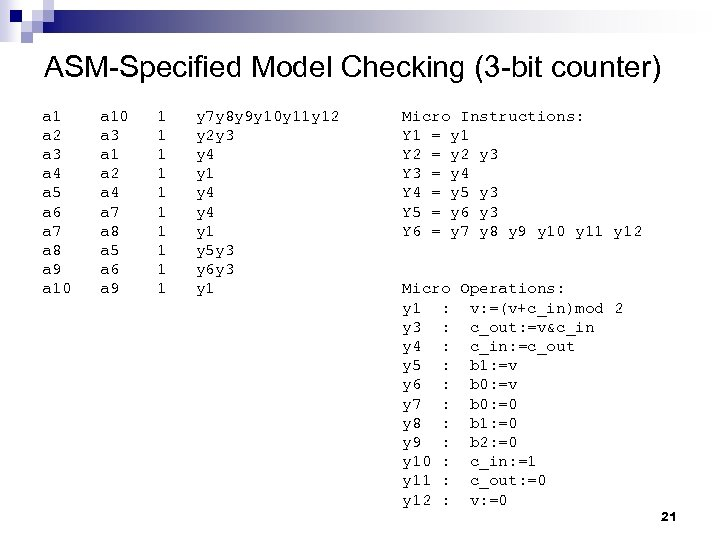ASM-Specified Model Checking (3 -bit counter) a 1 a 2 a 3 a 4 a 5 a 6 a 7 a 8 a 9 a 10 a 3 a 1 a 2 a 4 a 7 a 8 a 5 a 6 a 9 1 1 1 1 1 y 7 y 8 y 9 y 10 y 11 y 12 y 2 y 3 y 4 y 1 y 5 y 3 y 6 y 3 y 1 Micro Instructions: Y 1 = y 1 Y 2 = y 2 y 3 Y 3 = y 4 Y 4 = y 5 y 3 Y 5 = y 6 y 3 Y 6 = y 7 y 8 y 9 y 10 y 11 y 12 Micro Operations: y 1 : v: =(v+c_in)mod 2 y 3 : c_out: =v&c_in y 4 : c_in: =c_out y 5 : b 1: =v y 6 : b 0: =v y 7 : b 0: =0 y 8 : b 1: =0 y 9 : b 2: =0 y 10 : c_in: =1 y 11 : c_out: =0 y 12 : v: =0 21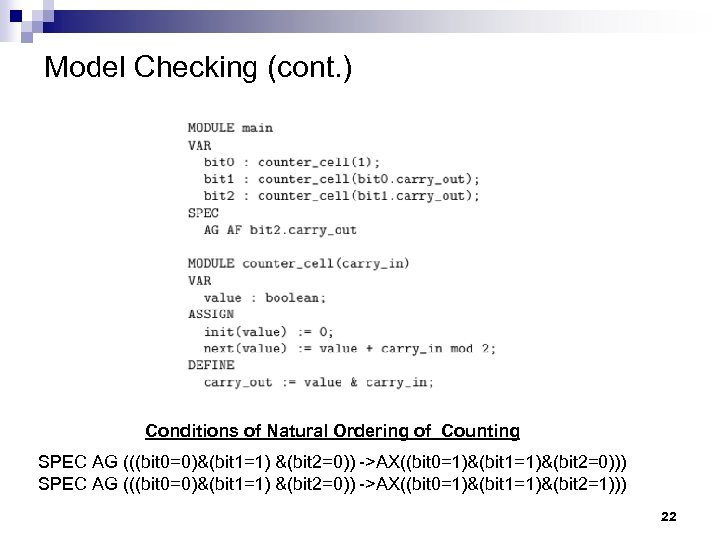Model Checking (cont. ) Conditions of Natural Ordering of Counting SPEC AG (((bit 0=0)&(bit 1=1) &(bit 2=0)) ->AX((bit 0=1)&(bit 1=1)&(bit 2=0))) SPEC AG (((bit 0=0)&(bit 1=1) &(bit 2=0)) ->AX((bit 0=1)&(bit 1=1)&(bit 2=1))) 22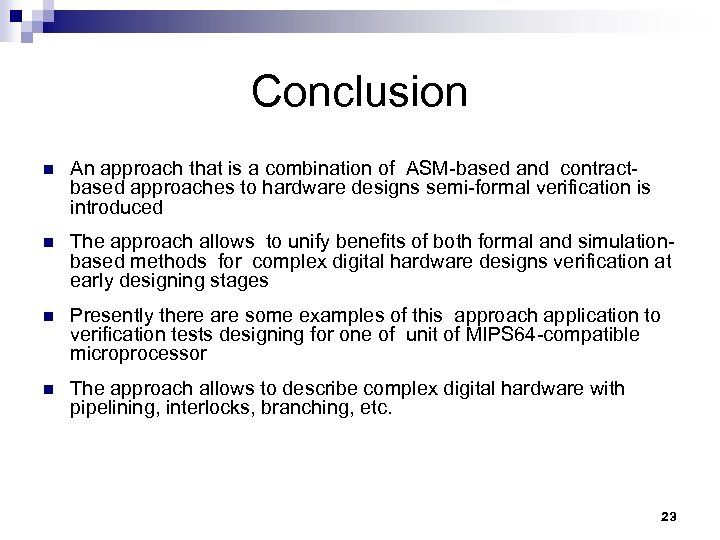Conclusion n An approach that is a combination of ASM-based and contractbased approaches to hardware designs semi-formal verification is introduced n The approach allows to unify benefits of both formal and simulationbased methods for complex digital hardware designs verification at early designing stages n Presently there are some examples of this approach application to verification tests designing for one of unit of MIPS 64 -compatible microprocessor n The approach allows to describe complex digital hardware with pipelining, interlocks, branching, etc. 23Thank You! 24